Topic 8: Imperfect Competition

# Solutions: Diamond’s Demise

1. As a monopolist, what is the total effect of a price change from $2,400 to$1,600 on revenue? Break this change into an increase and a decrease.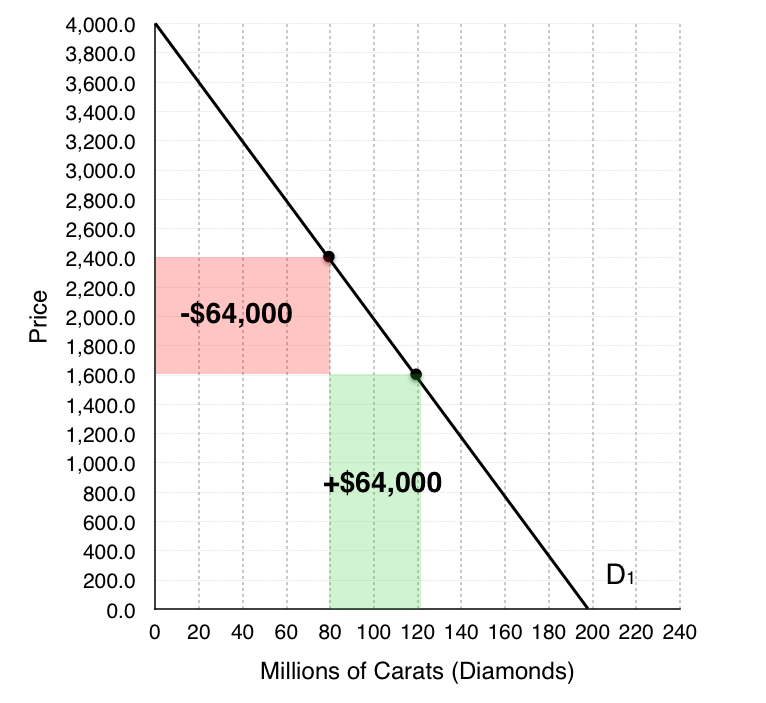Based on our demand curve, when DeBeers decreases its prices two things happen – DeBeers loses money on the 80 million Carats of diamonds they were originally selling, but they also gain from 40 million new Carats they are able to sell. Calculating the areas of the increase and the decreases, we find that they are both equal to $64,000. 2. As a monopolist, what quantity does DeBeers produce? What price do they charge?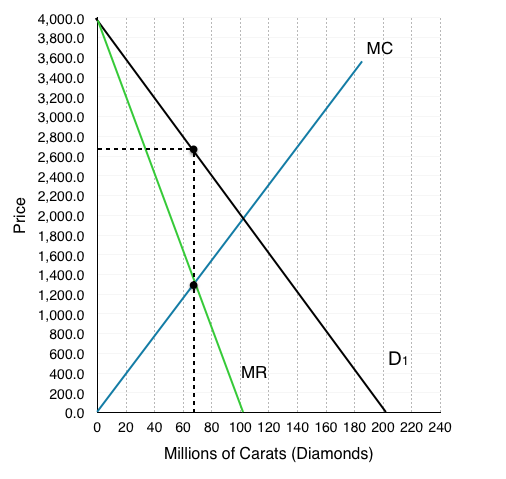A monopolist charges the highest price possible where MR = MC. Here, that occurs at a quantity of 65 million Carats and a price of$2,700.

3. What is Consumer Surplus, Producer Surplus, and Market Surplus?

We can do both number 3 and 4 together on the graph below. Calculating the areas:

Monopoly (graph on right)

Producer Surplus (yellow area):

(2,700 – 1,300)(65) + $\frac{\left(1300-0\right)\left(65\right)}{2}$

=$133.25 billion Consumer Surplus (blue area): $\frac{\left(4000-2700\right)\left(65\right)}{2}$ =$42.25 billion

Market Surplus (blue + yellow):

$133.25 billion +$42.25 billion

=$175.5 billion 4. What is the deadweight loss under monopoly? Remember that social surplus is maximized where MC = MB, or where our MC intersects the demand curve. This occurs at a quantity of 100 million carats and a price of$2,000

Social Surplus Maximizing (graph on left)

Producer Surplus (yellow area):

$\frac{\left(2000-0\right)\left(100\right)}{2}$

=$100 billion Consumer Surplus (blue area):$latex \frac{\left(4000-2000\right)\left(100\right)}{2}$=$100 billion

Market Surplus (blue + yellow):

$100 billion +$100 billion

=$200 billion The difference between the market surplus under monopoly, and the market surplus when the market is maximizing social surplus is equal to the deadweight loss. Note that social surplus could also be maximized under perfect price discrimination. DWL =$200 billion- $175.5 billion =$24.5 billion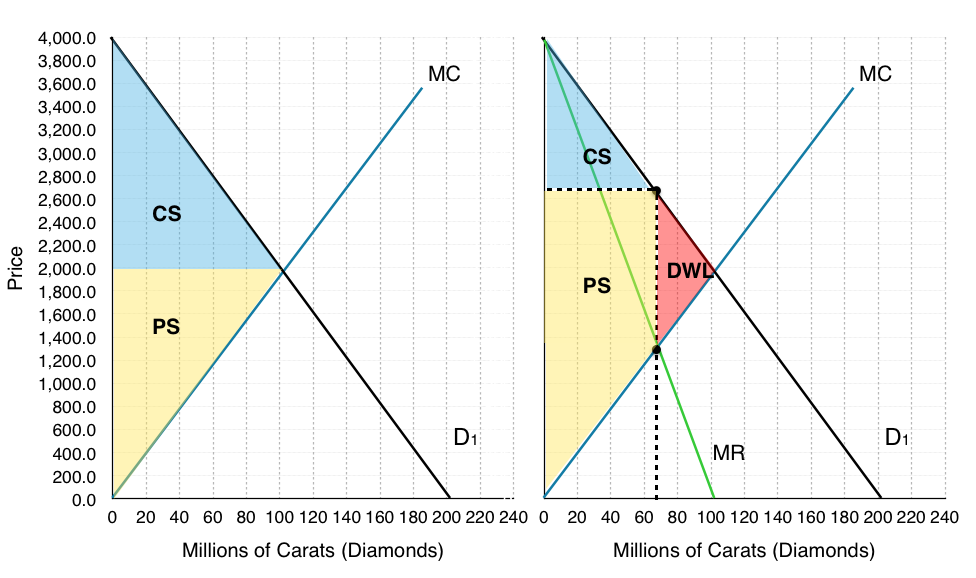5. What are DeBeers profits? Why are they able to sustain these in the long-run?

To calculate DeBeers profits we must find the ATC @ our given quantity. Since we know that P-ATC = profit per unit, we can calculate the rectangular area between these two points to find profit. At quantity of 65 million Carats of diamonds, our ATC = $1,700. ($2,700 – $1,700)(65) =$65 billion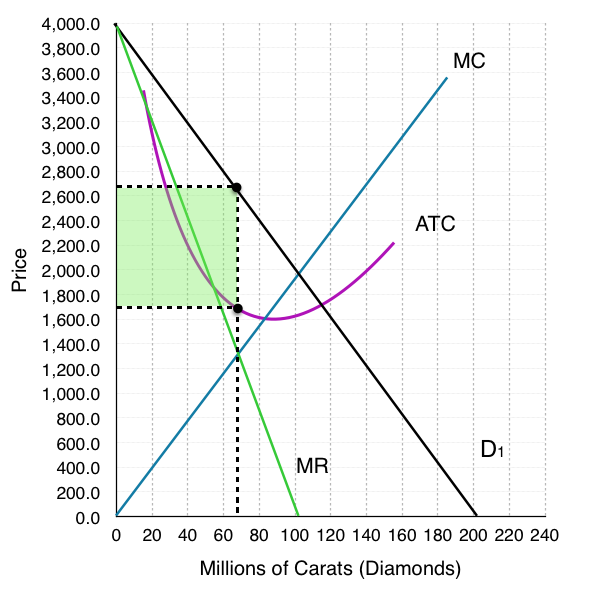6. Show the effect of the changes on our demand curve in the long run. If the new marginal revenue intersects marginal cost at (45, 1,900) draw the new demand curve and new marginal revenue curve.

As shown in the figure below as more firms enter the market, our demand curve no longer represents the demand curve for the entire market. Some of DeBeer’s consumers will go elsewhere, and the demand curve that DeBeer’s faces will shift inwards. As long as there are positive profits (P > ATC) then this process will continue to occur. As we can see at point (45, $1900) our demand curve (D2) is tangent to the ATC curve and MR = MC. These are the conditions for a monopolistic competitor. 7. As a monopolistic competitor, what quantity does DeBeers produce? What price do they charge? Similar to a monopoly, a monopolistic competitor charges the highest price possible where MR = MC. Here, that occurs at a quantity of 45 million Carats and a price of$1,900.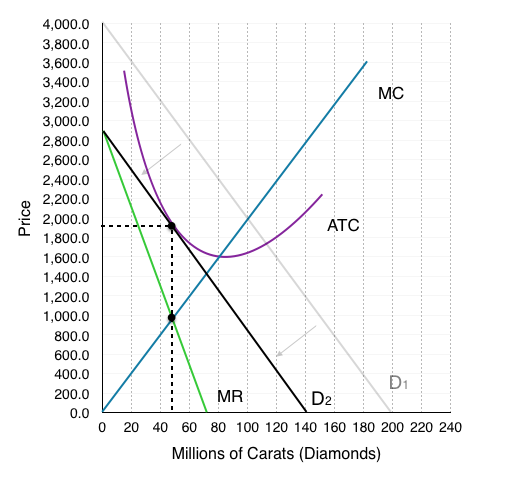8. What are DeBeers profits now?

Remember that under monopolistic competition, profits are 0 in the long-run. Since the diamond market has reached a state of long-run equilibrium, De Beers profits will be 0. In the diagram above we can see that P=ATC, meaning that there are no profits.

9. What is the deadweight loss under monopolistic competition?

Remember that social surplus is maximized where MC = MB, or where our MC intersects the demand curve. This occurs at a quantity of 75 million carats and a price of $1,400. We could once again calculate the consumer, and producer surplus, or we could also note on the diagram that the decrease in surplus is equal to the red shaded area. Calculating the red area: ($2,000 – $1,000) ( 70 – 45 ) /2 =$17,500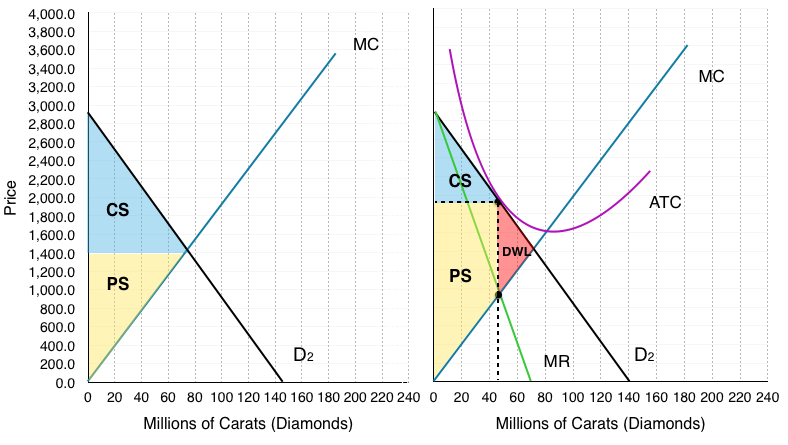10. Are these market changes good or bad for consumers?

We actually can’t find directly the changes in consumer surplus by the information we are given, since we cannot compare the deadweight loss from questions 4 and question 9. This is because both of these look at the individual firm, and for monopolistic competition, we know there are other firms in the market. We can notice that our price has decreased, and that our drop quantity demanded will be offset by supply from other firms. We also know that consumers generally prefer variety, and by opening up market more options are available.